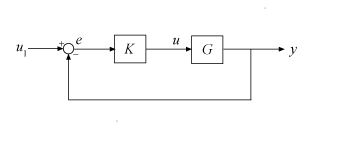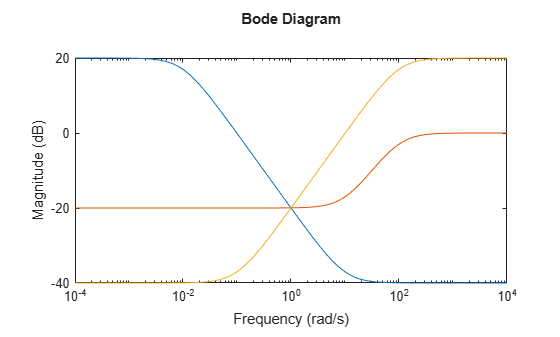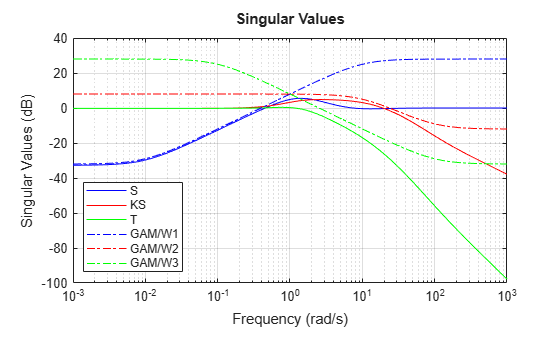mixsyn

Mixed-sensitivity H synthesis method for robust control loop-shaping design

Description

example

[K,CL,gamma,info] = mixsyn(G,W1,W2,W3) computes a controller that minimizes the H norm of the weighted closed-loop transfer function

$M\left(s\right)=\left[\begin{array}{c}{W}_{1}S\\ {W}_{2}KS\\ {W}_{3}T\end{array}\right],$

where S = (I + GK)–1 and T = (IS) is the complementary sensitivity of the following control system.You choose the weighting functions W1,W2,W3 to shape the frequency responses for tracking and disturbance rejection, controller effort, and noise reduction and robustness, respectively. For details about how to choose weighting functions, see Mixed-Sensitivity Loop Shaping.

mixsyn computes the controller K that yields the minimum ||M(s)||, which is returned as gamma. For the returned controller K,

$\begin{array}{c}{‖S‖}_{\infty }\le \gamma |{W}_{1}^{-1}|\\ {‖KS‖}_{\infty }\le \gamma |{W}_{2}^{-1}|\\ {‖T‖}_{\infty }\le \gamma |{W}_{3}^{-1}|.\end{array}$

[K,CL,gamma] = mixsyn(G,W1,W2,W3,gamTry) calculates a controller for the target performance level gamTry. Specifying gamTry can be useful when the optimal controller performance is better than you need for your application. In that case, a less-than-optimal controller can have smaller gains and be better conditioned numerically. When W1,W2,W3 capture the desired limits on the gains of S, KS, and T, use gamtry = 1 to just enforce those limits.

If gamTry is not achievable, mixsyn returns [] for K and CL, and Inf for gamma.

[K,CL,gamma] = mixsyn(G,W1,W2,W3,gamRange) searches the range gamRange for the best achievable performance. Specify the range with a vector of the form [gmin,gmax]. Limiting the search range can speed up computation by reducing the number of iterations performed by mixsyn to test different performance levels.

[K,CL,gamma] = mixsyn(___,opts) specifies additional computation options. To create opts, use hinfsynOptions. Specify opts after all other input arguments.

[K,CL,gamma,info] = mixsyn(___) returns a structure containing additional information about the H synthesis computation. You can use this argument with any of the previous syntaxes.

Examples

collapse all

Use mixsyn for sensitivity and complementary sensitivity loop shaping. Create a plant model and weighting functions that:

• Shape the sensitivity function for reference tracking and disturbance rejection (W1 = 1/S large inside the control bandwidth).

• Shape the complementary sensitivity for robustness and noise attenuation (W3 = 1/T large outside the control bandwidth).

• Limit the control effort (W2 = 1/KS large inside the control bandwidth).

s = zpk('s');
G = (s-1)/(s+1)^2;

W1 = makeweight(10,[1 0.1],0.01);
W2 = makeweight(0.1,[32 0.32],1);
W3 = makeweight(0.01,[1 0.1],10);

bodemag(W1,W2,W3)Design the controller.

[K,CL,gamma] = mixsyn(G,W1,W2,W3);

mixsyn shapes the singular values of the sensitivity function S, the complementary sensitivity function T, and the control effort R = K*S. Examine the results of the synthesis and the shapes of these transfer functions.

S = feedback(1,G*K);
KS = K*S;
T = 1-S;
sigma(S,'b',KS,'r',T,'g',gamma/W1,'b-.',ss(gamma/W2),'r-.',gamma/W3,'g-.',{1e-3,1e3})
legend('S','KS','T','GAM/W1','GAM/W2','GAM/W3','Location','SouthWest')
gridInput Arguments

collapse all

Plant, specified as a dynamic system model such as a state-space (ss) model. G can be any LTI model. mixsyn assumes the following control structure.If G is a generalized state-space model with uncertain or tunable control design blocks, then mixsyn uses the nominal or current value of those elements.

Weighting functions, specified as dynamic system models. Choose the weighting functions W1,W2,W3 to shape the frequency responses for tracking and disturbance rejection, controller effort, and noise reduction and robustness. Typically:

• For good reference-tracking and disturbance-rejection performance, choose W1 large inside the control bandwidth to obtain small S.

• For robustness and noise attenuation, choose W3 large outside the control bandwidth to obtain small T.

• To limit control effort in a particular frequency band, increase the magnitude of W2 in this frequency band to obtain small KS.

If one of the weights is not needed, set it to []. For instance, if you do not want to restrict control effort, use W2 = [].

Use makeweight to create weighting functions with the desired gain profiles. For details about choosing weighting functions, see Mixed-Sensitivity Loop Shaping.

If G has NU inputs and NY outputs, then W1,W2,W3 must be either SISO or square systems of size NY, NU, and NY, respectively.

Because S + T = I, mixsyn cannot make both S and T small (less than 0 dB) in the same frequency range. Therefore, when you specify weights for loop shaping, there must be a frequency band in which both W1 and W3 are below 0 dB.

Target performance level, specified as a positive scalar. mixsyn attempts to compute a controller such that the H of the weighted closed-loop system M(s) does not exceed gamTry. If this performance level is achievable, then the returned controller has gammagamTry. If gamTry is not achievable, mixsyn returns an empty controller.

Performance range for search, specified as a vector of the form [gmin,gmax]. The mixsyn command tests only performance levels within that range. It returns a controller with performance:

• gammagmin, when gmin is achievable.

• gmin < gamma < gmax, when gmax is achievable and but gmin is not.

• gamma = Inf when gmax is not achievable. In this case, mixsyn returns [] for K and CL.

If you know a range of feasible performance levels, specifying this range can speed up computation by reducing the number of iterations performed by mixsyn to test different performance levels.

Additional options for the computation, specified as an options object you create using hinfsynOptions.

Use opts to specify options for the underlying hinfsyn computation (see Algorithms). Available options include:

• Display algorithm progress at the command line.

• Turn off automatic scaling and regularization.

• Specify an optimization method.

For information about all options, see hinfsynOptions.

Output Arguments

collapse all

Controller, returned as a state-space (ss) model object or [].

If you supply gamTry or gamRange and the specified performance values are not achievable, then K = [].

Augmented closed-loop transfer function, returned as a state-space (ss) model object or []. The augmented closed-loop transfer function is given by

$M\left(s\right)=\left[\begin{array}{c}{W}_{1}S\\ {W}_{2}KS\\ {W}_{3}T\end{array}\right],$

where S = (I + GK)–1 and T = (IS) is the complementary sensitivity of the unweighted control system. See Mixed-Sensitivity Loop Shaping.

The returned performance level gamma is the H norm of CL.

If you supply gamTry or gamRange and the specified performance levels are not achievable, then CL = [].

Controller performance, returned as a nonnegative scalar value or Inf. This value is the performance achieved using the returned controller K, and is the H norm of CL (see hinfnorm). If you do not provide performance levels to test using gamTry or gamRange, then gamma is the best achievable performance level.

If you provide gamTry or gamRange, then gamma is the actual performance level achieved by the controller computed for the best passing performance level that hinfsyn tries. If the specified performance levels are not achievable, then gamma = Inf.

Additional synthesis data, returned as a structure or [] (if the specified performance level is not achievable). info contains data about the underlying hinfsyn computation used by mixsyn to minimize the H norm of the closed-loop transfer function M(s). For details about the meaning of the fields of info, see the info output argument of hinfsyn.

Algorithms

mixsyn uses your weighting functions to generate an augmented plant P = augw(G,W1,W2,W3). It then invokes hinfsyn to find a controller that minimizes the H norm of the closed-loop transfer function M(s) = LFT(P,K). For details, see Mixed-Sensitivity Loop Shaping.

Compatibility Considerations

expand all

Not recommended starting in R2019b

Behavior changed in R2019b